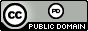시간 제한 메모리 제한 제출 정답 맞은 사람 정답 비율
1 초 1024 MB 9 8 6 100.000%

## 문제

Hill encryption (devised by mathematician Lester S. Hill in 1929) is a technique that makes use of matrices and modular arithmetic.  It is ideally used with an alphabet that has a prime number of characters, so we'll use the $37$ character alphabet A, B, $\ldots$, Z, 0, 1, $\ldots$, 9, and the space character.  The steps involved are the following:

1. Replace each character in the initial text (the plaintext) with the substitution A$\rightarrow 0$, B$\rightarrow 1$, $\ldots$, (space)$\rightarrow 36$.  If the plaintext is ATTACK AT DAWN this becomes $$0\ 19\ 19\ 0\ 2\ 10\ 36\ 0\ 19\ 36\ 3\ 0\ 22\ 13$$.
2. Group these number into three-component vectors, padding with spaces at the end if necessary.  After this step we have $\left( \begin{array}{c} 0 \\ 19 \\ 19 \end{array} \right) \left( \begin{array}{c} 0 \\ 2 \\ 10 \end{array} \right) \left( \begin{array}{c} 36 \\ 0 \\ 19 \end{array} \right) \left( \begin{array}{c} 36 \\ 3 \\ 0 \end{array} \right) \left( \begin{array}{c} 22 \\ 13 \\ 36 \end{array} \right)$
3. Multiply each of these vectors by a predetermined $3 \times 3$ encryption matrix using modulo $37$ arithmetic. If the encryption matrix is $\left( \begin{array}{ccc} 30 & 1 & 9 \\ 4 & 23 & 7 \\ 5 & 9 & 13 \end{array} \right)$ then the first vector is transformed as follows: $\begin{eqnarray*} \left( \begin{array}{ccc} 30 & 1 & 9 \\ 4 & 23 & 7 \\ 5 & 9 & 13 \end{array} \right) \left( \begin{array}{c} 0 \\ 19 \\ 19 \end{array} \right) & = & \left( \begin{array}{c} (30 \times 0 + 1 \times 19 + 9 \times 19) \mod 37 \\ (4 \times 0 + 23 \times 19 + 7 \times 19) \mod 37 \\ (5 \times 0 + 9 \times 19 + 13 \times 19) \mod 37 \end{array} \right) \\ & = & \left( \begin{array}{c} 5 \\ 15 \\ 11 \end{array} \right) \end{eqnarray*}$
4. After multiplying all the vectors by the encryption matrix, convert the resulting values back to the $37$-character alphabet and concatenate the results to obtain the encrypted ciphertext.  In our example the ciphertext is FPLSFA4SUK2W9K3.

This method can be generalized to work with any $n \times n$ encryption matrix in which case the initial plaintext is broken up into vectors of length $n$.  For this problem you will be given an encryption matrix and a plaintext and must compute the corresponding ciphertext.

## 입력

Input begins with a line containing a positive integer $n \leq 10$ indicating the size of the matrix and the vectors to use in the encryption.  After this are $n$ lines each containing $n$ non-negative integers specifying the encryption matrix. After this is a single line containing the plaintext consisting only of characters in the $37$-character alphabet specified above.

## 출력

Output the corresponding ciphertext on a single line.

## 예제 입력 1

3
30 1 9
4 23 7
5 9 13
ATTACK AT DAWN


## 예제 출력 1

FPLSFA4SUK2W9K3


## 예제 입력 2

6
26 11 23 14 13 16
6 7 32 4 29 29
26 19 30 10 30 11
6 28 23 5 24 23
6 24 1 27 24 20
13 9 32 18 20 18
MY HOVERCRAFT IS FULL OF EELS


## 예제 출력 2

W4QVBO0NJG5 Y76H5A6XHR11BV670Z


## 출처• 문제를 만든 사람: Bob Roos